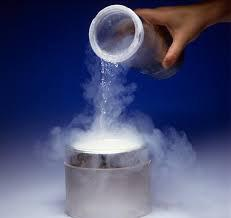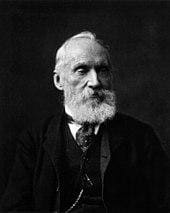# Do You Know- Reaching Absolute Zero Temperature is as difficult as attaining Speed of LightWhat is the lowest temperature that you have experienced? Our body temperature is 37 degree Celsius. Room temperature is lower than that which is 25 degree Celsius. Fridges are generally at four degree Celsius and ice forms at zero degree Celsius. Can temperature go below zero degree Celsius? Yes. Temperatures below zero degree Celsius are generally measured in Kelvin scale, where 273 K is equal to zero degree Celsius. Temperature of liquid nitrogen is approximately 70 Kelvin and liquid helium is approximately 4 kelvin. As we decrease the temperature, there are many curious phenomena that happen which have significant effects on properties of materials. Zero kelvin or -273.15 degree Celsius temperature is known as absolute zero temperature.
Robert Boyle was the first person to discuss the existence of an absolute minimal temperature. In his book “New Experiments and Observations touching Cold” written in 1665, he introduced the concept of Primum Frigidum which is absolute pure cold. French physicist, Guillaume Amontons, improved the air-thermometer and came up with a lower limit to the temperature in 1972. He proposed that the boiling-point of water is +73 and the melting-point of ice is 51, so that the zero is about −240 on the Celsius scale and this was a close approximation to the present value of −273.15 °C. Further improvements by Johann Heinrich Lambert in 1779 proposed that −270 °C might be regarded as absolute cold. Lord Kelvin devised a scale of absolute temperature in 1848, which was independent of the properties of any particular substance and his observation was based on Carnot’s theory of the Motive Power of Heat and Henri Victor Regnault’s experiments.

Later, Kelvin scale was constructed where its zero was placed at −273 °C. Now, Absolute zero of temperature is the point at which a thermodynamic system has the lowest energy. It is −273.15 °C on the Celsius temperature scale and −459.67 °F on the Fahrenheit temperature scale.It was observed that gases tend to contract indefinitely as the temperature reduces. This behavior of gases at low pressures suggested that there could be an ultimate low temperature at which an ideal gas would reach zero volume at constant pressure. This temperature is now referred to as an absolute zero of temperature. The third law of thermodynamics is stated as follows: “The entropy of a perfect crystal at absolute zero is exactly equal to zero”. At absolute zero of temperature, the system must be with the minimum possible energy. The concept of absolute zero has many thermodynamic consequences. All the molecular motion does not cease at absolute zero but no energy from molecular motion is available for transfer to other systems, and it is therefore correct to say that the energy at absolute zero is minimal.
Temperature is a measure of the amount of internal, which is a randomized energy in a material. As temperature decreases , entropy or disorder and the random motions of the molecules in the material decreases. According to classical Physics absolute zero is defined as the point at which these molecular motions stop entirely. But according to quantum mechanics it is not correct to say that molecules are not moving at absolute zero. Quantum mechanics helps us to study all the possible states of some system with the use of wave functions. For every system there are many states with different energy. At absolute zero temperature, the system is sitting in that lowest energy state. As the temperature increases, the system tends to spend more time in higher energy states.
Reaching to absolute zero is as difficult as attaining speed of light. Scientists have cooled atoms to amazingly low temperatures close to absolute zero. The current record is about 450 Pico Kelvin and it was achieved by researchers at MIT in 2003. To achieve a velocity which is equal to the speed of light requires an infinite amount of work, and for decreasing temperature to absolute zero requires extracting an infinite amount of heat. So it is impossible to achieve both.